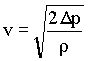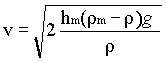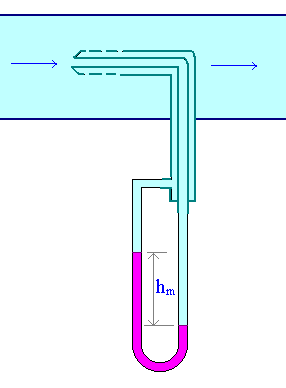### Pitot Tube

Home -> Lecture Notes -> Fluid Mechanics -> Unit-IIIThe pitot tube is a device to measure the local velocity along a streamline. The pitot tube has two tubes: one is static tube(b), and another is impact tube(a). The opening of the impact tube is perpendicular to the flow direction. The opening of the static tube is parallel to the direction of flow. The two legs are connected to the legs of a manometer or equivalent device for measuring small pressure differences. The static tube measures the static pressure, since there is no velocity component perpendicular to its opening. The impact tube measures both the static pressure and impact pressure (due to kinetic energy). In terms of heads the impact tube measures the static pressure head plus the velocity head.

The reading (hm) of the manometer will therefore measure the velocity head, and

v2/2g = Pressure head measured indicated by the pressure measuring device

i.e. v2/2 = Dp/rà 1

Pressure difference indicated by the manometer Dp is given by,

Dp = hm(rm - r)gPitot tube - A convenient setup:It consists of two concentric tubes arranged parallel to the direction of flow; the impact pressure is measured on the open end of the inner tube. The end of the outer concentric tube is sealed and a series of orifices on the curved surface give an accurate indication of the static pressure. For the flow rate not to be appreciably disturbed, the diameter of the instrument must not exceed about one fifth of the diameter of the pipe. An accurate measurement of the impact pressure can be obtained using a tube of very small diameter with its open end at right angles to the direction of flow; hypodermic tubing is convenient for this purpose.

The pitot tube measures the velocity of only a filament of liquid, and hence it can be used for exploring the velocity distribution across the pipe cross-section. If, however, it is desired to measure the total flow of fluid through the pipe, the velocity must be measured at various distance from the walls and the results integrated. The total flow rate can be calculated from a single reading only of the velocity distribution across the cross-section is already known.

A perfect pitot tube should obey equn.1 exactly, but all actual instruments must be calibrated and a correction factor applied.